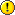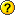## Prediction-Realization Diagram

If you are seeking RATS code for implementing a particular technique or replicating results from a paper, post your request here. Be sure to include complete citations for any papers or books.

### Prediction-Realization Diagram

Hi Tom,

A request: can we have a procedure for Henri Theil's Prediction-Realization Diagram to identify change/turning points?

There's also an extension from Jarrell & Stanley(1992) A note on diagrams for turning-point diagnostics, Journal for Forecasting, 11(4), 325-330

many thanks,
Amarjit
ac_1

Posts: 296
Joined: Thu Apr 15, 2010 6:30 am
Location: London, UK

### Re: Prediction-Realization Diagram

Hi Tom,

Maybe have the diagram as part of the @UForeErrors procedure.

Also, it would be good to have a 45-degrees line and a percentage in each quadrant.

Cheers,
Amarjit
ac_1

Posts: 296
Joined: Thu Apr 15, 2010 6:30 am
Location: London, UK

### Re: Prediction-Realization Diagram

The LINES option does straight lines in scatter plots. GRTEXT can put whatever text you want into a scatter plot.
TomDoan

Posts: 7388
Joined: Wed Nov 01, 2006 5:36 pm

### Re: Prediction-Realization Diagram

Hi Tom,

Further to the above there is:
FILIZ et al (2021) Sticky Stock Market Analysts, Journal of Risk and Financial Management, 2021, 14, 593, pp.1-27.
https://www.econstor.eu/bitstream/10419/258696/1/jrfm-14-00593-v2.pdf

and in:
ELTON GRUBER BROWN GOETZMANN (2014) Modern Portfolio Theory and Investment Management, 9th Edn, chapter 27, p.703-p.711.

Here's code to generate a prediction-realization diagram:
Code: Select all
`set(first=-3.0) xx 1954:07 2004:07 = xx{1}+0.01; * 45-degree-line, xx==yy lineprin / xxset yy 1954:07 2004:07 = xx; * 45-degree-line, xx==yy linespgraphscatter(style=symbols,overlay=line,ovsame,\$         header="Prediction Realization Diagram\\Dynamic multi-12-step ahead forecasts 2020:05 2021:04",\$         hlabel="Forecast Change\\(y-axis)",hscale=both,\$         vlabel="Actual Change\\(x-axis)",vscale=both,\$         hmax=+3.0,hmin=-3.0,vmax=+3.0,vmin=-3.0) 2# Dr yhat_D_dyfa end-11 end 20# xx yy 1954:07 2004:07 2grtext(x=+3.0,y=+3.0,size=30,align=right) "Line of Perfect\\Forecasts"grtext(x=+0.01,y=+2.15,align=left) "QUADRANT 1-O\\Actual Positive Change\\is OverEstimated\\[CORRECT SIGN]"grtext(x=+2.98,y=+0.10,align=right) "QUADRANT 1-U\\Actual Positive Change\\is UnderEstimated\\[CORRECT SIGN]"grtext(x=-2.98,y=+2.15,align=left) "QUADRANT 2\\Forecast Positive Change\\Actual Negative Change\\[WRONG SIGN, TURNING POINT]"grtext(x=-0.01,y=-2.80,align=right) "QUADRANT 3-O\\Actual Negative Change\\is OverEstimated\\[CORRECT SIGN]"grtext(x=-2.99,y=-0.90,align=left) "QUADRANT 3-U\\Actual Negative Change\\is UnderEstimated\\[CORRECT SIGN]"grtext(x=+2.99,y=-2.80,align=right) "QUADRANT 4\\Forecast Negative Change\\Actual Positive Change\\[WRONG SIGN, TURNING POINT]"spgraph(done)`

The problem's are:
- it is 'hard-coded'
- the 45 degrees-line is as a long series of points
- I am not sure how to use the LINES option?
- I can only plot the 45 degrees-line in a square diagram.

Is there a way to automate the process given the differences in the actual 'Dr' and the differences between actual and forecasts 'yhat_D_dyfa'?

Ideally I'd like a procedureInterestingly, on a square prediction-realization diagram, while the differences between successive actual values (the Dr series) can vary naturally as per series i.e. points dotted all the way from left-to-right horizontally, the differences between successive actual and forecasts (the yhat_D_dyfa series) can be clustered around zero vertically, i.e. there's consistent differences, regardless of the levels, between successive forecasts and actuals; both for dynamic (anchored from h-steps back) and static (both the difference series being from 1-step back) forecasts. Thus there's a 'horizontal-line' of points all the way from left-to-right on the scatter-graph. The only reason I can think of why this may be is that forecasts are conditional expectations, and as such the difference between the actuals and forecasts represent an average or consistent expectation. Correctthanks,
Amarjit
ac_1

Posts: 296
Joined: Thu Apr 15, 2010 6:30 am
Location: London, UK